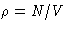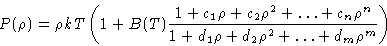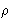# Problems of Liquids Theory

Problem of Virial Expansion:
Virial expansion is a polynomial in density. It is a continuous non-singular function of the density. We know however, that the density cannot exceed the value of the closed packing 0.74, therefore the equation of state should diverge. Empirical recipe: let's cook some divergent function and fit it with the known behavior at low densities.The expansion at smallshould coincide with virial expansion! If we know N=n+m virial coefficients, (n,m)-Padé approximant can be constructed. (3,3) approximant describes the simulation (P,V,T) data for hard spheres a lot better, than the corresponding virial expansion with 6 virial coefficients. This is however empirical and ambiguous approach. Alternative description is necessary.